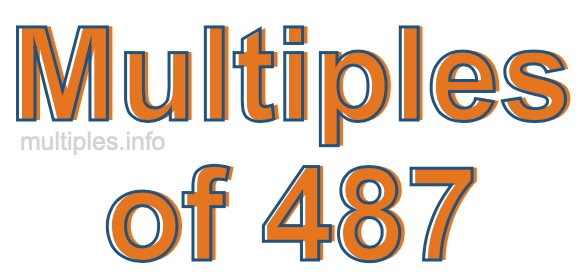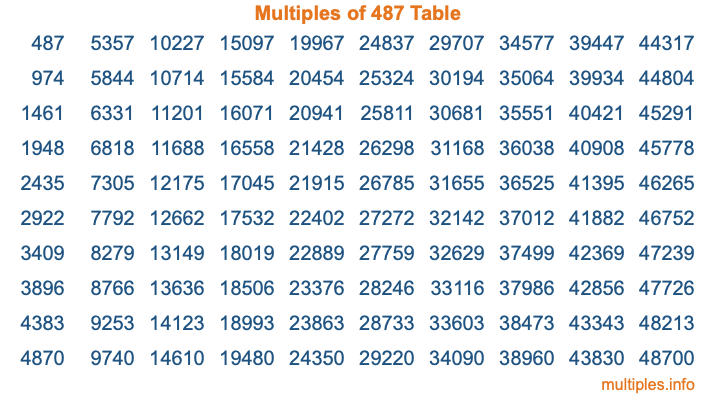Multiples of 487Welcome to the Multiples of 487 page. Here we will first teach you everything you will ever need to know about the multiples of 487, and then give you a study guide summary of everything we taught you to make sure you remember it all. Use this page to look up facts and learn information about the multiples of 487. This page will make you a multiples of four hundred eighty-seven expert!

Definition of Multiples of 487
Multiples of 487 are all the numbers that when divided by 487 equal an integer. Each of the multiples of 487 are called a multiple. A multiple of 487 is created by multiplying 487 by an integer.

Therefore, to create a list of multiples of 487, you start with 1 multiplied by 487, then 2 multiplied by 487, then 3 multiplied by 487, and so on for as long as you want. Thus, the list of the first five multiples of 487 is 487, 974, 1461, 1948, and 2435. To see a larger list of multiples of 487, see the printable image of Multiples of 487 further down on this page. We also have a category where you can choose any nth multiple of 487.

Multiples of 487 Checker
The Multiples of 487 Checker below checks to see if any number of your choice is a multiple of 487. In other words, it checks to see if there is any number (integer) that when multiplied by 487 will equal your number. To do that, we divide your number by 487. If the the quotient is an integer, then your number is a multiple of 487.

Is  a multiple of 487?

Least Common Multiple of 487 and ...
A Least Common Multiple (LCM) is the lowest multiple that two or more numbers have in common. This is also called the smallest common multiple or lowest common multiple and is useful to know when you are adding our subtracting fractions. Enter one or more numbers below (487 is already entered) to find the LCM.

Check out our LCM Calculator if you need more details about the Least Common Multiple or if you need the LCM for different numbers for adding and subtraction fractions.

nth Multiple of 487
As we stated above, 487 is the first multiple of 487, 974 is the second multiple of 487, 1461 is the third multiple of 487, and so on. Enter a number below to find the nth multiple of 487.

th multiple of 487

Multiples of 487 vs Factors of 487
487 is a multiple of 487 and a factor of 487, but that is where the similarities end. All postive multiples of 487 are 487 or greater than 487. All positive factors of 487 are 487 or less than 487.

Below is the beginning list of multiples of 487 and the factors of 487 so you can compare:

Multiples of 487: 487, 974, 1461, 1948, 2435, etc.

Factors of 487: 1, 487

As you can see, the multiples of 487 are all the numbers that you can divide by 487 to get a whole number. The factors of 487, on the other hand, are all the whole numbers that you can multiply by another whole number to get 487.

It's also interesting to note that if a number (x) is a factor of 487, then 487 will also be a multiple of that number (x).

Multiples of 487 vs Divisors of 487
The divisors of 487 are all the integers that 487 can be divided by evenly. Below is a list of the divisors of 487.

Divisors of 487: 1, 487

The interesting thing to note here is that if you take any multiple of 487 and divide it by a divisor of 487, you will see that the quotient is an integer.

Multiples of 487 Table
Below is an image of the first 100 multiples of 487 in a table. The table is in chronological order, column by column. The first column has the first ten multiples of 487, the second column has the next ten multiples of 487, and so on.The Multiples of 487 Table is also referred to as the 487 Times Table or Times Table of 487. You are welcome to print out our table for your studies.

Negative Multiples of 487
Although not often discussed or needed in math, it is worth mentioning that you can make a list of negative multiples of 487 by multiplying 487 by -1, then by -2, then by -3, and so on, to get the following list of negative multiples of 487:

-487, -974, -1461, -1948, -2435, etc.

Multiples of 487 Summary
Below is a summary of important Multiples of 487 facts that we have discussed on this page. To retain the knowledge on this page, we recommend that you read through the summary and explain to yourself or a study partner why they hold true.

There are an infinite number of multiples of 487.

A multiple of 487 divided by 487 will equal a whole number.

487 divided by a factor of 487 equals a divisor of 487.

The nth multiple of 487 is n times 487.

The largest factor of 487 is equal to the first positive multiple of 487.

487 is a multiple of every factor of 487.

487 is a multiple of 487.

A multiple of 487 divided by a divisor of 487 equals an integer.

487 divided by a divisor of 487 equals a factor of 487.

Any integer times 487 will equal a multiple of 487.

Multiples of a Number
Here you can get the multiples of another number, all with the same attention to detail as we did for multiples of 487 on this page.

Multiples of
Multiples of 488
Did you find our page about multiples of four hundred eighty-seven educational? Do you want more knowledge? Check out the multiples of the next number on our list!